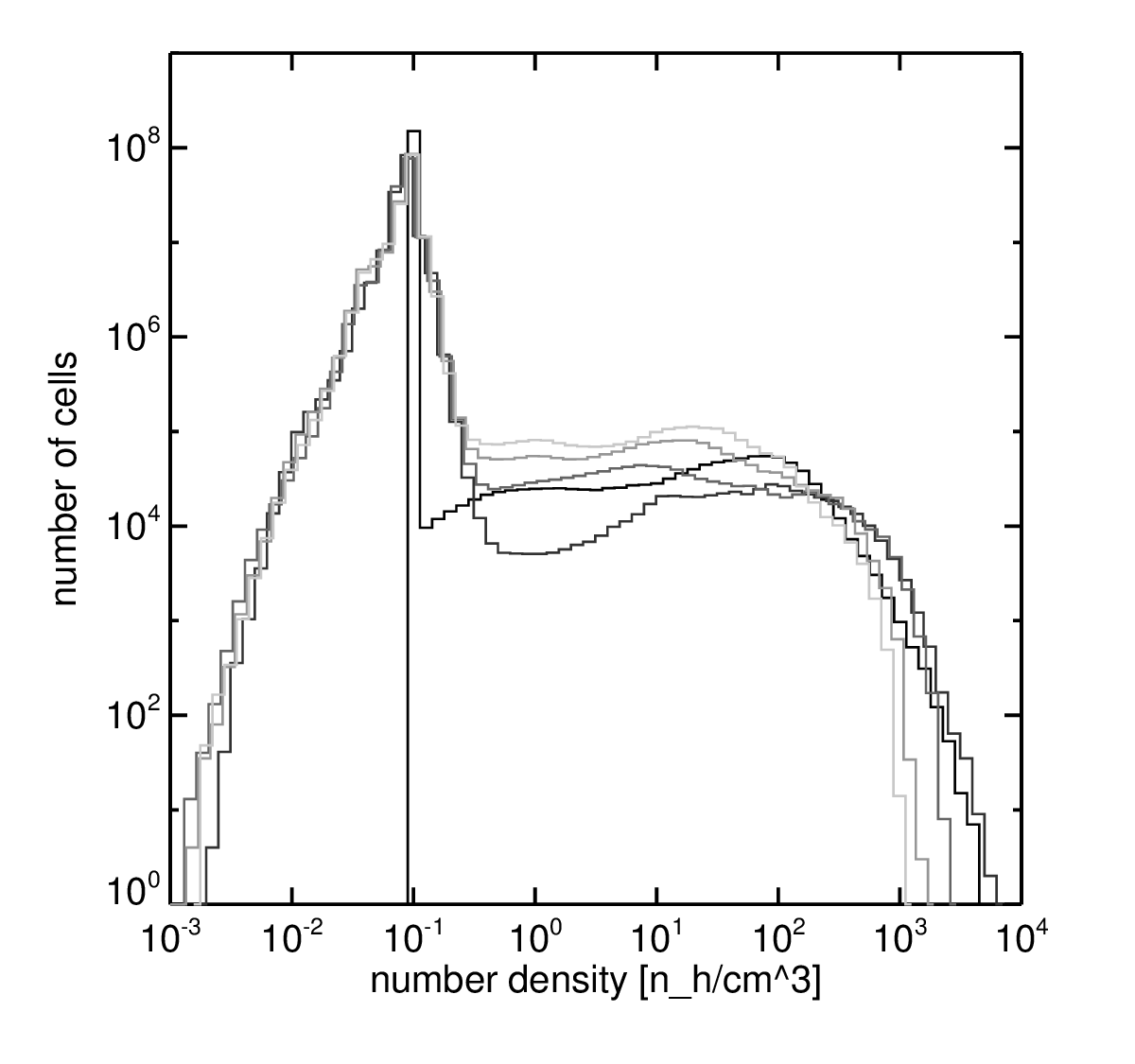# Cloud density evolution

## December 7, 2015

The following images and plot are products from a cloud-wind simulation with the following parameters:

• Resolution: 1024x384x384
• Size: 80x30x30 pc
• Wind density: $n_{wind} = 0.1 \, \mathrm{cm}^{-3}$
• Wind temperature: $T_{wind} = 5 \times 10^6 \, \mathrm{K}$
• Wind velocity: $v_{wind} = 1200 \, \mathrm{km} \, \mathrm{s}^{-1}$
• Average cloud density: $n_{cloud} \approx 75 \, \mathrm{cm}^{-3}$
• Cloud mass: $\approx 800 \, \mathrm{M}_{\odot}$
All material with a number density higher than $n = 1.0$ is considered part of the cloud.